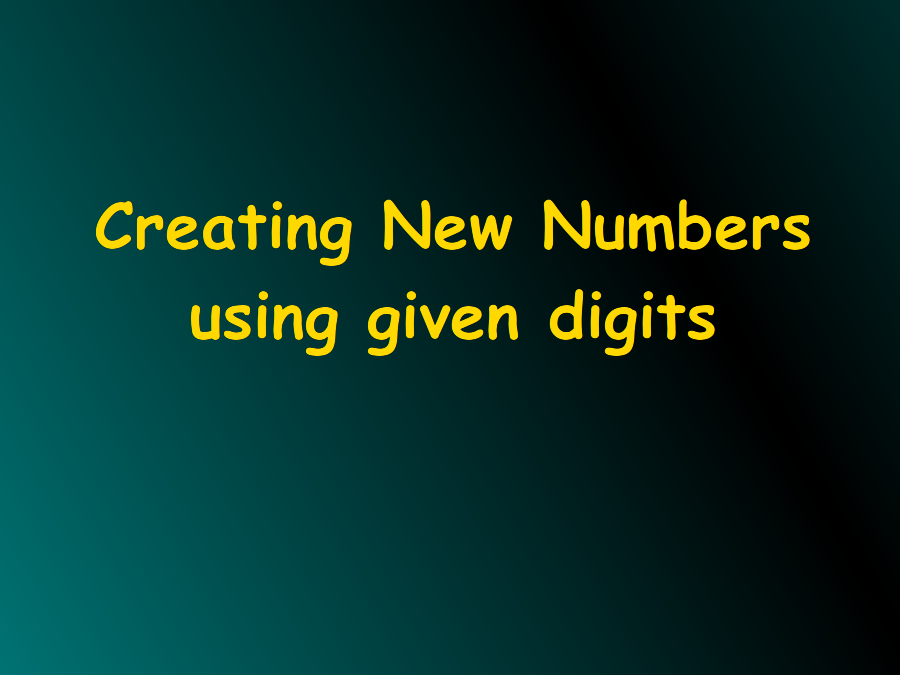#### Understanding Large Numbers

Predecessor and Successor, Formation and application of large Numbers Formation of numbers from digits.

#### Learner's Guide

Objectives:

• Addition, subtraction, multiplication, and division for a banker
• Predecessor and Successor
• Formation of Big Number
• Formation of numbers from digit
• Use of Large Numbers in Daily Life Situations

-------------------------------

Predecessor and Successor

1/4What is the predecessor of 19078?

1. 19077
2. 19078
3. 19079
4. 20077

19077

Predecessor = Number - 1

= 19078 - 1 = 19077

What is the biggest 2 digit number?

99

What is the smallest 3 digit number?

100

Successor of number 99 is __________________

99+1 = 100

--------------------------------

Formation of Big Number and their usage

How many thousands are there in 1 lakh?

 Lakh ten thousand thousand hundred ten one 1 lakh 1 0 0 0 0 0 1 thousand 1 0 0 0

1 lakh = 100000

1 thousand = 1000

1 lakh / 1 thousand = 100000/1000 = 100

1 lakh = 100 x 1 thousand

Formation of numbers from given digits:

How many digits are there in number: 2398

1. 1
2. 2
3. 3
4. 5
5. 4

4

 2 3 9 8

Make different 2 digit numbers using digits: 2 and 9

 Ten One Number 2 9 29 9 2 92 If repetition of numbers is allowed 2 2 22 9 9 99

1/6#### Exercise

• How many numbers can you form using digits: 2, 2?

Difficulty Level: Easy

Single digit numbers:

• 2

2 digit numbers:

• 22
• What is the successor of 9999?

1. 10000
2. 1000
3. 1000
4. 9990
Difficulty Level: Easy

10000

• Number of 10s in 1 lakh = ______________

Difficulty Level: Moderate

1 lakh = 1 00 000

so number of 10s = = 10 000